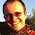## Friday, April 19, 2013

### Computing the Strahler numbers for nodes on a tree

Can anyone suggest an easy way of determining Strahler numbers of nodes on a given phylogenetic tree (see http://en.wikipedia.org/wiki/Strahler_number for details).

This should be possible with a simple post-order tree traversal, which we can do easily by reordering our tree "pruningwise" using reorder.phylo(...,"pruningwise") from the ape package.

I think the following code does this, but I'm going to ask the poster to check this for me:

strahlerNumber<-function(tree,plot=TRUE){
cw<-reorder(tree,"pruningwise")
oo<-sapply(tree\$edge[,2],function(x,y)
which(x==y),y=cw\$edge[,2])
SS<-matrix(0,nrow(cw\$edge),2)
SS[cw\$edge[,2]<=length(cw\$tip.label),2]<-1
nn<-unique(cw\$edge[,1])
for(i in 1:cw\$Nnode){
jj<-which(cw\$edge[,1]==nn[i])
s<-sort(SS[jj,2],decreasing=TRUE)
SS[jj,1]<-if(all(sapply(s[2:length(s)],"<",s))) s
else s+1
SS[which(cw\$edge[,2]==cw\$edge[jj,1]),2]<-SS[jj,1]
}
ss<-setNames(c(SS[oo,][1,1],SS[oo,2]),
c(tree\$edge[1,1],tree\$edge[,2]))
ss<-ss[order(as.numeric(names(ss)))]
names(ss)[1:length(tree\$tip.label)]<-tree\$tip.label
if(plot){
plot(tree,no.margin=TRUE,edge.width=2)
nodelabels(ss[1:tree\$Nnode+length(tree\$tip.label)])
}
return(ss)
}

E.g.,

> tree<-pbtree(n=12)
> ss<-strahlerNumber(tree,plot=TRUE)
> ss
t11 t12  t3  t2  t5  t6  t4  t9 t10  t7  t8  t1
1  1  1  1  1  1  1  1  1  1  1  1
13  14  15  16  17  18  19  20  21  22  23
3  3  2  2  3  3  2  3  2  2  2

1.Hi Liam,

Alastair here (the r-sig-phylo subscriber that asked this question).

Wowsers. This solution works amazingly well, and is far more elegant to my (still slightly wrong) code. Thanks very much!

I have an additional question, if I may: how could I go about extracting clades of a certain order as unique groups?

For example, if I wanted to extract all the 2nd order clades in the example figure above there would be four groups:
t8,t7
t10,t9,t4
t5,t6
t11,t12,t3

I've been trying to extract the groups based solely on the assigned Strahler number for each node, but the lower ranking identical numbers mess with the extraction (i.e create unique clades that are just subsets of a bigger clade of the same order).

Thanks for time and amazing R skills.

Cheers,
Alastair

1.No problem.